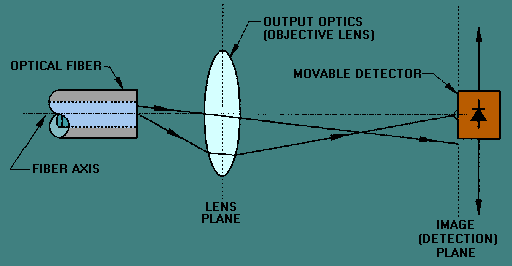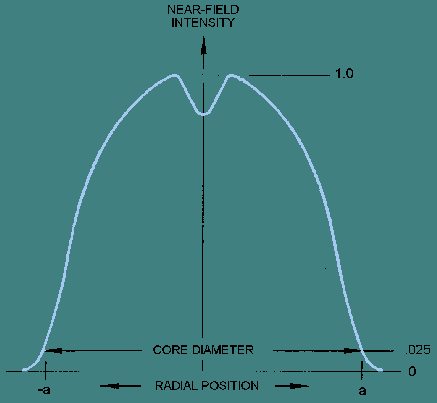radiation pattern. Our discussion is limited to measuring the core diameter directly from the output near-field radiation pattern obtained using EIA/TIA-455-43. The near-field power distribution is defined as the emitted power per unit area (radiance) for each position in the plane of the emitting surface. "> Core DiameterCustom SearchCore Diameter Core diameter is measured using EIA/TIA-455-58. The core diameter is defined from the refractive index profile n(r) or the output near-field radiation pattern. Our discussion is limited to measuring the core diameter directly from the output near-field radiation pattern obtained using EIA/TIA-455-43. The near-field power distribution is defined as the emitted power per unit area (radiance) for each position in the plane of the emitting surface. For this chapter, the emitting surface is the output area of a fiber-end face. Near-field power distributions describe the emitted power per unit area in the near-field region. The near-field region is the region close to the fiber-end face. In the near-field region, the distance between the fiber-end face and detector is in the micrometers (μm) range. EIA/TIA-455-43 describes the procedure for measuring the near-field power distribution of optical waveguides. Output optics, such as lenses, magnify the fiber-end face and focus the fiber's image on a movable detector. Figure 5-8 shows an example setup for measuring the near-field power distribution. The image is scanned in a plane by the movable detector. The image may also be scanned by using a detector array. Detector arrays of known element size and spacing may provide a display of the power distribution on a video monitor. A record of the near-field power is kept as a function of scan position. Figure 5-8. - The measurement of the near-field power distribution.The core diameter (D) is derived from the normalized output near-field radiation pattern. The normalized near-field pattern is plotted as a function of radial position on the fiber-end face. Figure 5-9 shows a plot of the normalized near-field radiation pattern as a function of scan position. Figure 5-9. - Near-field radiation pattern.The core diameter (D) is defined as the diameter at which the intensity is 2.5 percent of the maximum intensity (see figure 5-9). The 2.5 percent points, or the 0.025 level, intersects the normalized curve at radial positions -a and a. The core diameter is simply equal to 2a (D=2a). Q.17 Near-field power distributions describe the emitted power per unit area in the near-field region. Describe the near-field region. Q.18 How is the core diameter defined?Integrated Publishing, Inc. - A (SDVOSB) Service Disabled Veteran Owned Small Business